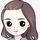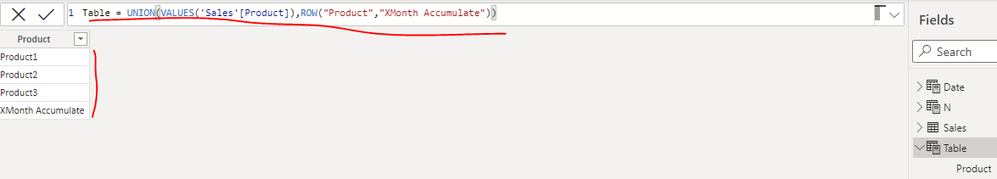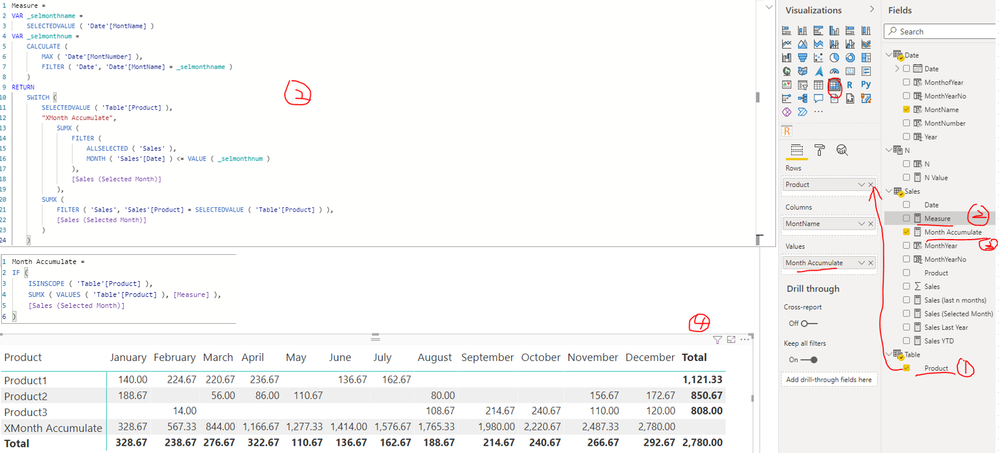cancel
Showing results for
Did you mean:Resolver I

## Add new row with accumulate in Matrix

Hi,

It's possible add a new a row in Matrix with the Month Accumulate?
I have the total and i would like a new row with the accumulate.

Like this example:My Example file:

Thank you.

1 ACCEPTED SOLUTIONCommunity Support

I updated your sample pbix file(see attachment), please check whether that is what you want.

1. Create a calculated table with below formula

``Table = UNION(VALUES('Sales'[Product]),ROW("Product","XMonth Accumulate"))``2. Create two measures as below to get the sum of sales

``````Measure =
VAR _selmonthname =
SELECTEDVALUE ( 'Date'[MontName] )
VAR _selmonthnum =
CALCULATE (
MAX ( 'Date'[MontNumber] ),
FILTER ( 'Date', 'Date'[MontName] = _selmonthname )
)
RETURN
SWITCH (
SELECTEDVALUE ( 'Table'[Product] ),
"XMonth Accumulate",
SUMX (
FILTER (
ALLSELECTED ( 'Sales' ),
MONTH ( 'Sales'[Date] ) <= VALUE ( _selmonthnum )
),
[Sales (Selected Month)]
),
SUMX (
FILTER ( 'Sales', 'Sales'[Product] = SELECTEDVALUE ( 'Table'[Product] ) ),
[Sales (Selected Month)]
)
)``````
``````Month Accumulate =
IF (
ISINSCOPE ( 'Table'[Product] ),
SUMX ( VALUES ( 'Table'[Product] ), [Measure] ),
[Sales (Selected Month)]
)``````

3. Create a matrix visual (Rows: field Product of Table   Column: field MonthName in Date table  Values: [Month Accumulate] )Best Regards

Community Support Team _ Rena
If this post helps, then please consider Accept it as the solution to help the other members find it more quickly.
4 REPLIES 4Community Support

I updated your sample pbix file(see attachment), please check whether that is what you want.

1. Create a calculated table with below formula

``Table = UNION(VALUES('Sales'[Product]),ROW("Product","XMonth Accumulate"))``2. Create two measures as below to get the sum of sales

``````Measure =
VAR _selmonthname =
SELECTEDVALUE ( 'Date'[MontName] )
VAR _selmonthnum =
CALCULATE (
MAX ( 'Date'[MontNumber] ),
FILTER ( 'Date', 'Date'[MontName] = _selmonthname )
)
RETURN
SWITCH (
SELECTEDVALUE ( 'Table'[Product] ),
"XMonth Accumulate",
SUMX (
FILTER (
ALLSELECTED ( 'Sales' ),
MONTH ( 'Sales'[Date] ) <= VALUE ( _selmonthnum )
),
[Sales (Selected Month)]
),
SUMX (
FILTER ( 'Sales', 'Sales'[Product] = SELECTEDVALUE ( 'Table'[Product] ) ),
[Sales (Selected Month)]
)
)``````
``````Month Accumulate =
IF (
ISINSCOPE ( 'Table'[Product] ),
SUMX ( VALUES ( 'Table'[Product] ), [Measure] ),
[Sales (Selected Month)]
)``````

3. Create a matrix visual (Rows: field Product of Table   Column: field MonthName in Date table  Values: [Month Accumulate] )Best Regards

Community Support Team _ Rena
If this post helps, then please consider Accept it as the solution to help the other members find it more quickly.Resolver I

Hi, it's work like a charm!
Thank so much!Super User

Simple enough, but first rectify the date table,

``Date = CALENDAR("1/1/2016", "12/31/2018")``Thanks to the great efforts by MS engineers to simplify syntax of DAX! Most beginners are SUCCESSFULLY MISLED to think that they could easily master DAX; but it turns out that the intricacy of the most frequently used RANKX() is still way beyond their comprehension! DAX is simple, but NOT EASY!Super User